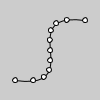Name

bezierPoint()

ExamplesnoFill()
bezier(85, 20, 10, 10, 90, 90, 15, 80)
fill(255)
steps = 10
for i in range(steps+1):
t = i / float(steps)
x = bezierPoint(85, 10, 90, 15, t)
y = bezierPoint(20, 10, 90, 80, t)
ellipse(x, y, 5, 5)
Description Evaluates the Bezier at point t for points a, b, c, d. The parameter t varies between 0 and 1, a and d are points on the curve, and b and c are the control points. This can be done once with the x coordinates and a second time with the y coordinates to get the location of a bezier curve at t.
Syntax
bezierPoint(a, b, c, d, t)
Parameters
a float: coordinate of first point on the curve float: coordinate of first control point float: coordinate of second control point float: coordinate of second point on the curve float: value between 0 and 1
Related bezier()
bezierVertex()
curvePoint()

Updated on Mon Sep 21 15:53:24 2020.

If you see any errors or have comments, please let us know.